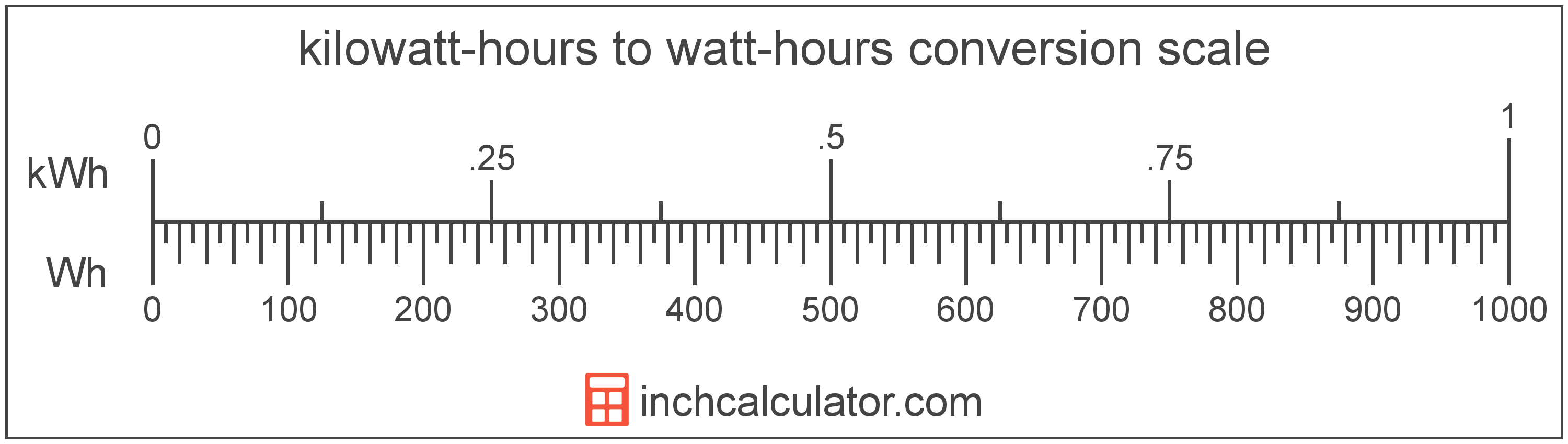# Watt-Hours to Kilowatt-Hours Converter

Enter the energy in watt-hours below to get the value converted to kilowatt-hours.

Results in Kilowatt-Hours:1 Wh = 0.001 kWh

Do you want to convert kilowatt-hours to watt-hours?

## How to Convert Watt-Hours to Kilowatt-Hours

To convert a measurement in watt-hours to a measurement in kilowatt-hours, divide the energy by the following conversion ratio: 1,000 watt-hours/kilowatt-hour.

Since one kilowatt-hour is equal to 1,000 watt-hours, you can use this simple formula to convert:

kilowatt-hours = watt-hours ÷ 1,000

The energy in kilowatt-hours is equal to the energy in watt-hours divided by 1,000.

For example, here's how to convert 500 watt-hours to kilowatt-hours using the formula above.
kilowatt-hours = (500 Wh ÷ 1,000) = 0.5 kWh## What Is a Watt-Hour?

The watt-hour is a measure of electrical energy equal to one watt of power over a one hour period.

Watt-hours are usually abbreviated as Wh, although the formally adopted expression is W·h. The abbreviation W h is also sometimes used. For example, 1 watt-hour can be written as 1 Wh, 1 W·h, or 1 W h.

In formal expressions, the centered dot (·) or space is used to separate units used to indicate multiplication in an expression and to avoid conflicting prefixes being misinterpreted as a unit symbol.

## What Is a Kilowatt-Hour?

A kilowatt-hour is a measure of electrical energy equal to one kilowatt, or 1,000 watts, of power over a one hour period. Kilowatt-hours are a measure of electrical work performed over a period of time, and are often used as a way of measuring energy usage by electric companies.

Kilowatt-hours are usually abbreviated as kWh, although the formally adopted expression is kW·h. The abbreviation kW h is also sometimes used. For example, 1 kilowatt-hour can be written as 1 kWh, 1 kW·h, or 1 kW h.

## Watt-Hour to Kilowatt-Hour Conversion Table

Table showing various watt-hour measurements converted to kilowatt-hours.
Watt-hours Kilowatt-hours
1 Wh 0.001 kWh
2 Wh 0.002 kWh
3 Wh 0.003 kWh
4 Wh 0.004 kWh
5 Wh 0.005 kWh
6 Wh 0.006 kWh
7 Wh 0.007 kWh
8 Wh 0.008 kWh
9 Wh 0.009 kWh
10 Wh 0.01 kWh
20 Wh 0.02 kWh
30 Wh 0.03 kWh
40 Wh 0.04 kWh
50 Wh 0.05 kWh
60 Wh 0.06 kWh
70 Wh 0.07 kWh
80 Wh 0.08 kWh
90 Wh 0.09 kWh
100 Wh 0.1 kWh
200 Wh 0.2 kWh
300 Wh 0.3 kWh
400 Wh 0.4 kWh
500 Wh 0.5 kWh
600 Wh 0.6 kWh
700 Wh 0.7 kWh
800 Wh 0.8 kWh
900 Wh 0.9 kWh
1,000 Wh 1 kWh

## References

1. Bureau International des Poids et Mesures, The International System of Units (SI), 9th edition, 2019, https://www.bipm.org/documents/20126/41483022/SI-Brochure-9-EN.pdf